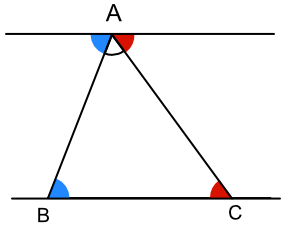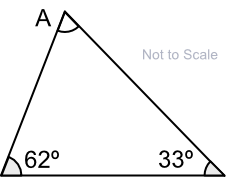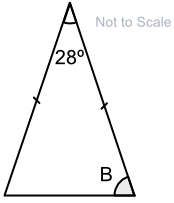Angles in a Triangle

## Angles in a Triangle

A triangle has three sides and three angles.

The angles in a triangle always add up to 180º.

Higher students: the number of degrees in a triangle can be derived using Alternate Angles:

Angles at A and B (blue) are alternate angles;

Angles at A and C (red) are alternate angles;

Angles at A (blue, white and red) are on a straight line = 180º;

Therefore the number of degrees in a triangle is 180.## Example 1

What is the size of angle A?There are 180º in a triangle. Subtract the other angles from 180 for the answer: 180 - 62 - 33 = 85º

## Example 2

What is the size of angle B?It is an Isosceles triangle. Two sides of the triangle are the same length (shown by the marks on two of the lines); therefore two angles also have the same value.

The angle that is given is the odd one out. Subtract this from 180º: 180 - 28 = 152º. Divide this by 2 for the value of B: 152 ÷ 2 = 76º